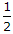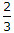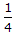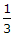# Civil Engineering - Theory of Structures - Discussion

Discussion Forum : Theory of Structures - Section 4 (Q.No. 19)
19.
The ratio of the length and depth of a simply supported rectangular beam which experiences maximum bending stress equal to tensile stress, due to same load at its mid span, isExplanation:
No answer description is available. Let's discuss.
Discussion:
6 comments Page 1 of 1.

Priya said:   6 months ago
Why moment is taken as wl/4? Please explain to me.

Snigdha said:   4 years ago
Thank you @Asay.

Asay said:   6 years ago
Bending Stress=M(max)/Z
=(PL/4)/(I/y)
=(PL/4)/[(bd^3/12)/(d/2)]
=(PL/4)/[bd^2/6]
=3PL/2bd^2
Now,
Tensile Stress=P/A
=P/bd.

Since, according to question,
Bending Stress=Tensile Stress
PL/2bd^2=P/bd.
====> L/d=2/3

So, B is the correct Answer.
(1)

Sumit said:   6 years ago
Z is the moment of inertia or what?
(1)

Chhaya said:   6 years ago
Thanks @Ranjit.

Ranjit ray said:   7 years ago
Mmax = wl/4.
Bending stress = M/z
Tensile stress = w/bd
z = bd2/6.
By solving & equating we will get the answer.